UPSC  >  Olympiad Test: Squares And Square Roots

# Olympiad Test: Squares And Square Roots - UPSC

Test Description

## 40 Questions MCQ Test CSAT Preparation - Olympiad Test: Squares And Square Roots

Olympiad Test: Squares And Square Roots for UPSC 2023 is part of CSAT Preparation preparation. The Olympiad Test: Squares And Square Roots questions and answers have been prepared according to the UPSC exam syllabus.The Olympiad Test: Squares And Square Roots MCQs are made for UPSC 2023 Exam. Find important definitions, questions, notes, meanings, examples, exercises, MCQs and online tests for Olympiad Test: Squares And Square Roots below.
Solutions of Olympiad Test: Squares And Square Roots questions in English are available as part of our CSAT Preparation for UPSC & Olympiad Test: Squares And Square Roots solutions in Hindi for CSAT Preparation course. Download more important topics, notes, lectures and mock test series for UPSC Exam by signing up for free. Attempt Olympiad Test: Squares And Square Roots | 40 questions in 40 minutes | Mock test for UPSC preparation | Free important questions MCQ to study CSAT Preparation for UPSC Exam | Download free PDF with solutions
 1 Crore+ students have signed up on EduRev. Have you?
Olympiad Test: Squares And Square Roots - Question 1

### (8.01)2 + ? = (8.97)2 What will approximately come in place of question mark?

Detailed Solution for Olympiad Test: Squares And Square Roots - Question 1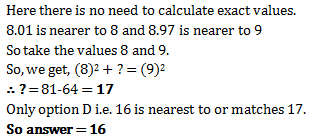Olympiad Test: Squares And Square Roots - Question 2

### The square of 23 is :

Detailed Solution for Olympiad Test: Squares And Square Roots - Question 2

Yes the correct option is A because..... 23×23=529.....

Olympiad Test: Squares And Square Roots - Question 3

### The square of which of the following would be even number?

Detailed Solution for Olympiad Test: Squares And Square Roots - Question 3

Since the square of an odd natural number is odd and that of an even number is an even number.

∴    (i)  The square of 431 is an odd number

(∵  431 is an odd number)

(ii)   The square of 2826 is an even nnumber.

(∵ 2826 is an even number)

(iii) The square of 7779 is an odd number

(∵ 7779 is an odd number)

(iv)  The square of 131 is an odd nnumber.

(∵ 82004 is an odd number)

Olympiad Test: Squares And Square Roots - Question 4

The square of which of the following would be odd number?

Detailed Solution for Olympiad Test: Squares And Square Roots - Question 4

Squares of odd numbers are always odd

Olympiad Test: Squares And Square Roots - Question 5

Which of the following is a perfect square ?

Detailed Solution for Olympiad Test: Squares And Square Roots - Question 5

81 is the square of 9..... 9 × 9 = 81

Olympiad Test: Squares And Square Roots - Question 6

What will be the one digit in the square of 1234

Detailed Solution for Olympiad Test: Squares And Square Roots - Question 6

One digit will be 6 because 1234
4, is at he last and 4² = 16.
So, 6 is at the last.

Olympiad Test: Squares And Square Roots - Question 7

What will be the number of zeros in the square of 400 ?

Detailed Solution for Olympiad Test: Squares And Square Roots - Question 7

Square of 400 = 160000

Therefore, the number of zeroes is 4

Olympiad Test: Squares And Square Roots - Question 8

The perfect square number between 30 and 40 is  :

Detailed Solution for Olympiad Test: Squares And Square Roots - Question 8

Since 35,32,39 are not perfect squares . hence the only perfect square between 30 and 40 is 36

Olympiad Test: Squares And Square Roots - Question 9

By what least number should we multiply 1008 to make it a perfect square?

Detailed Solution for Olympiad Test: Squares And Square Roots - Question 9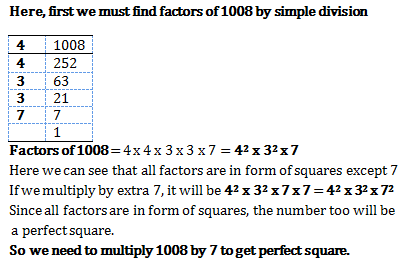Olympiad Test: Squares And Square Roots - Question 10

Sum of squares of two numbers is 145. If square root of one number is 3, find the other number.

Detailed Solution for Olympiad Test: Squares And Square Roots - Question 10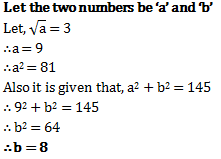Olympiad Test: Squares And Square Roots - Question 11

Which of the following number would have digit 1 at units place ?

Detailed Solution for Olympiad Test: Squares And Square Roots - Question 11

The squares that have 1 and 9 at their unit place, ends with 1.

IN this case, 81will end in 1

Olympiad Test: Squares And Square Roots - Question 12

How many natural numbers lie between 92 and 102?

Detailed Solution for Olympiad Test: Squares And Square Roots - Question 12

Between 92 and 102

Here, n = 9 and n + 1 =10

:. Natural number between 92 and 102  are (2 x n) or 2 x 9, i.e. 18. hence,correct option is (c).

Olympiad Test: Squares And Square Roots - Question 13

How many non square numbers lie between 11and 122?

Detailed Solution for Olympiad Test: Squares And Square Roots - Question 13

Between 112 and 122

Here, n = 11 and n + 1 = 12

∴ Natural numbers between 112 and 122 are (2 × n) or 2 × 11, i.e. 22.

Olympiad Test: Squares And Square Roots - Question 14

Which of the following are the factors of ac+ ab + bc + a2

Detailed Solution for Olympiad Test: Squares And Square Roots - Question 14

The question should be a2+ac+bc+ab
=a(a+c)+b(a+c)=(a+c)(a+b)

Olympiad Test: Squares And Square Roots - Question 15

How many numbers lie between square of 12 and 13?

Detailed Solution for Olympiad Test: Squares And Square Roots - Question 15

The number of non square numbers
between n² and ( n + 1 )² is 2n
Here,
n = 12,
n + 1 = 13
Therefore ,
Number of natural numbers lie
between 12² and 13² = 2 × 12
= 24

Olympiad Test: Squares And Square Roots - Question 16

Which of the following arc the factors of 1 – x2?

Olympiad Test: Squares And Square Roots - Question 17

What is the number of digits in the square root of 390625?

Detailed Solution for Olympiad Test: Squares And Square Roots - Question 17

Square root of 390625=625.Hence its a 3 digit number

Olympiad Test: Squares And Square Roots - Question 18

The square root of 6400 is

Detailed Solution for Olympiad Test: Squares And Square Roots - Question 18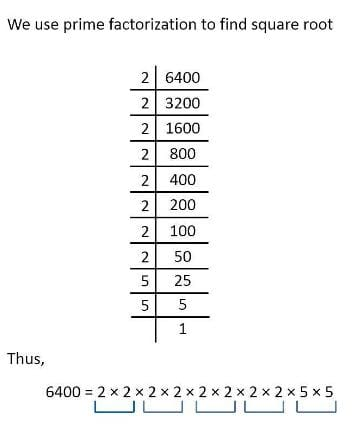Therefore, the square root is 80

Olympiad Test: Squares And Square Roots - Question 19

By which smallest number 90 must be multiplied so as to make it a perfect square ?

Detailed Solution for Olympiad Test: Squares And Square Roots - Question 19

By prime factorisation we have 90=2*3*3*5, so the least number by which 90 should be multiplied is 2*5=10

Olympiad Test: Squares And Square Roots - Question 20

By which smallest number 48 must be divided so as to make it a perfect square ?

Detailed Solution for Olympiad Test: Squares And Square Roots - Question 20

By Prime factorisation, we have 48=2*2*2*2*3. We have two pairs of 2 but no pair of 3. Hence 48 must be divided by 3 to make it a perfect square.

Olympiad Test: Squares And Square Roots - Question 21

Which smallest number should be added to 80 so as to make it a perfect square ?

Detailed Solution for Olympiad Test: Squares And Square Roots - Question 21

The smallest number to be added to 80 so as to obtain a perfect square number is 1.

80+1=81 and the square root of 81 is 9,so 81 becomes a perfect square.

Olympiad Test: Squares And Square Roots - Question 22

What could be the possible “one’s digit” of the square root of 625?

Detailed Solution for Olympiad Test: Squares And Square Roots - Question 22

625 is square root of 25 and one's digit of 25 is 5

Olympiad Test: Squares And Square Roots - Question 23

Which is the smallest three-digit perfect square?

Detailed Solution for Olympiad Test: Squares And Square Roots - Question 23

Yes as the the smallest 3 digit no. is 100 and it is the square of 10 so also a perfect square.

Olympiad Test: Squares And Square Roots - Question 24

Which is the greatest three-digit perfect square?

Detailed Solution for Olympiad Test: Squares And Square Roots - Question 24

We know that the largest 3 digit number is 999. So we will find out the square root of 999 by long division method and check whether it is a perfect square or not.
We will see that 999 is not a perfect square as it leaves 38 as remainder.

So if 38 will be added to 999 then it will become a 4 digit number. Therefore to find the largest 3 digit perfect square we will subtract 38 from 999.

999 - 38 = 961.

Hence 961 isthe largest 3 digit perfect square whose square root is 31.

Olympiad Test: Squares And Square Roots - Question 25

Which is the greatest 4-digit perfect square?

Detailed Solution for Olympiad Test: Squares And Square Roots - Question 25

By division method we find the root of 9999 and we get the remainder 198.So subtracting 198 from 9999 we get 9801 as the greatest 4-digit perfect square.

Olympiad Test: Squares And Square Roots - Question 26

Which is the smallest 4-digit perfect square?

Detailed Solution for Olympiad Test: Squares And Square Roots - Question 26

Apply division method on 1000, gives 3*3=9 leaves remainder 1 .Then get the two zeros, which makes it 100 then you find the next number ie,62*2=124 and then subtract 100 from 124 you get 24 which is to be added in 1000. Hence it’s 1024

Olympiad Test: Squares And Square Roots - Question 27

What will be the number of digits in the square root of 25600?

Detailed Solution for Olympiad Test: Squares And Square Roots - Question 27

The square root of 25600 is 160. Hence, number of digits is 3.

Olympiad Test: Squares And Square Roots - Question 28

What will be the number of digits in the square root of 1296?

Detailed Solution for Olympiad Test: Squares And Square Roots - Question 28

The square root of 1296 is 36.

Hence, number of digits os 2

Olympiad Test: Squares And Square Roots - Question 29

The square root of 12.25 is _______________ .

Detailed Solution for Olympiad Test: Squares And Square Roots - Question 29

The square root of 12.25 is 3.5

Olympiad Test: Squares And Square Roots - Question 30

What is the length of the side of a square whose area is 441 cm2 ?

Detailed Solution for Olympiad Test: Squares And Square Roots - Question 30

We know area of a square =a^2 given area =441cm^2 so a^2=441cm2 a^2=(21cm)^2 so a=21...length of square =21cm

Olympiad Test: Squares And Square Roots - Question 31

In a right angle triangle ABC, right angled at B, AB = 6cm, BC = 8cm ,then AC = ____ .

Detailed Solution for Olympiad Test: Squares And Square Roots - Question 31

We have (AB)2+(BC)=( AC)2 which is (AC)= 36+64 . Hence AC = 10cm.

Olympiad Test: Squares And Square Roots - Question 32

Which least number should be subtracted from 629 so as to get a perfect square ?

Detailed Solution for Olympiad Test: Squares And Square Roots - Question 32

If we subtract 4 from the given number, then it becomes 625.

625 is a square of 25.

Olympiad Test: Squares And Square Roots - Question 33

The square root of 1.21 is

Detailed Solution for Olympiad Test: Squares And Square Roots - Question 33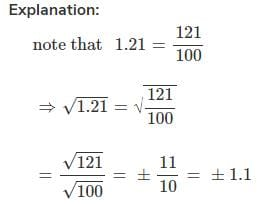Olympiad Test: Squares And Square Roots - Question 34

What is the smallest square number which is divisible by each of the numbers 6,9 and 15 ?

Detailed Solution for Olympiad Test: Squares And Square Roots - Question 34

LCM of 6,9 and 15 is 90. So any multiple of 90 will be divisible by 6,9 and 15. The only square number in the options is 900, so 900 is the smallest square number which is divisible by 6,9 and 15.

Olympiad Test: Squares And Square Roots - Question 35

The square of 1.2 is

Detailed Solution for Olympiad Test: Squares And Square Roots - Question 35

Required square of 1.2 is 1.44

Olympiad Test: Squares And Square Roots - Question 36

The square root of 169 is

Detailed Solution for Olympiad Test: Squares And Square Roots - Question 36

13 is the square root of 169.

Olympiad Test: Squares And Square Roots - Question 37

What is the length of the diagonal of a rectangle having dimensions 3 cm and 4 cm?

Detailed Solution for Olympiad Test: Squares And Square Roots - Question 37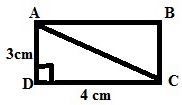Here let us consider ABCD is a rectangle given in the figure.

DC = 4 cm (Length)

To Find:

AC (Diagonal)

We know that, Triangle ADC is a right triangle, right angled at D. So by using Pythagorus Theorem

Length^2 + Breadth^2 = Hypotenuse^2     (diagonal)

=> 4^2 + 3^2 = AC^2

=> 16 + 9 = AC^2

=> 25 = AC^2

=> AC = √25 = 5

Therefore the length of the diagonal AC is 5 cm.

Olympiad Test: Squares And Square Roots - Question 38

What will be the length of third side of a right angled triangle whose hypotenuse is 5cm and one of the side is 3 cm ?

Detailed Solution for Olympiad Test: Squares And Square Roots - Question 38

By using Pythagoras theorem
H= P+ B2
Third side =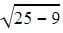= 4cm

Olympiad Test: Squares And Square Roots - Question 39

Which of the following is not a perfect square ?

Detailed Solution for Olympiad Test: Squares And Square Roots - Question 39

Squares of all integers are known as perfect squares. All perfect squares end in 1, 4, 5, 6, 9 or 00 (i.e. Even number of zeros). Therefore, a number that ends in 2, 3, 7 or 8 is not a perfect square.

Olympiad Test: Squares And Square Roots - Question 40

What is the difference between 1500 and nearest perfect square number smaller than 1500?

Detailed Solution for Olympiad Test: Squares And Square Roots - Question 40

We know that 402 = 1600

So we must start finding squares of numbers less than 40

Take 39 first

402 = 1600, :. 1600 -1500 = 100

392 = 1521,  :.1521 -1500 = 21

382= 1444, .: 1500 -1444 = 56

Thus nearest perfect square is 392.

But there is no option of difference of 21.

so we'll go the next available nearest possible that is 1444 i.e 382

So answer is 1500-1444 = 56

## CSAT Preparation

197 videos|151 docs|200 tests